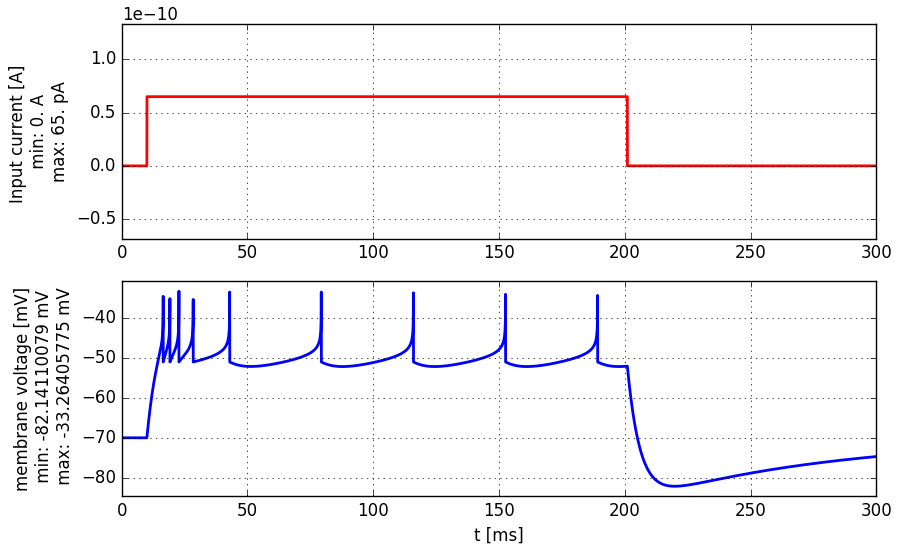Book chapters

The Adaptive Exponential Integrate-and-Fire model is introduced in Chapter 6 Section 1

Python classes

Use function AdEx.simulate_AdEx_neuron() to run the model for different input currents and different parameters. Get started by running the following script:

% matplotlib inline
import brian2 as b2
from neurodynex.tools import plot_tools, input_factory

current = input_factory.get_step_current(10, 250, 1. * b2.ms, 65.0 * b2.pA)
plot_tools.plot_voltage_and_current_traces(state_monitor, current)
print("nr of spikes: {}".format(spike_monitor.count))A step-current (top panel, red) is injected into an AdEx neuron. The membrane voltage of the neuron is shown in blue (bottom panel).

## 4.1. Exercise: Adaptation and firing patterns¶

We have implemented an Exponential Integrate-and-Fire model with a single adaptation current w:

(1)$\begin{split}\left[\begin{array}{ccll} \tau_m \frac{du}{dt}} &=& -(u-u_{rest}) + \Delta_T exp(\frac{u-\vartheta_{rh}}{\Delta_T}) - R w + R I(t) \\[.2cm] \tau_w \frac{dw}{dt}} &=& a (u-u_{rest}) -w + b \tau_w \sum_{t^{(f)}} \delta (t - t^{(f)}) \\[.2cm] \end{array}\right.\end{split$

### 4.1.1. Question: Firing pattern¶

• When you simulate the model with the default parameters, it produces the voltage trace shown above. Describe that firing pattern. Use the terminology of Fig. 6.1 in Chapter 6.1
• Call the function AdEx.simulate_AdEx_neuron() with different parameters and try to create adapting, bursting and irregular firing patterns. Table 6.1 in Chapter 6.1 provides a starting point for your explorations.
• In order to better understand the dynamics, it is useful to observe the joint evolution of u and w in a phase diagram. Use the function AdEx.plot_adex_state() to get more insights. Fig. 6.3 in Chapter 6 Section 2 shows a few trajectories in the phase diagram.

Note

If you want to set a parameter to 0, Brian still expects a unit. Therefore use a=0*b2.nS instead of a=0.

If you do not specify any parameter, the following default values are used:

MEMBRANE_TIME_SCALE_tau_m = 5 * b2.ms
MEMBRANE_RESISTANCE_R = 500*b2.Mohm
V_REST = -70.0 * b2.mV
V_RESET = -51.0 * b2.mV
RHEOBASE_THRESHOLD_v_rh = -50.0 * b2.mV
SHARPNESS_delta_T = 2.0 * b2.mV


## 4.2. Exercise: phase plane and nullclines¶

First, try to get some intuition on shape of nullclines by plotting or simply sketching them on a piece of paper and answering the following questions.

1. Plot or sketch the u- and w- nullclines of the AdEx model (I(t) = 0)
2. How do the nullclines change with respect to a?
3. How do the nullclines change if a constant current I(t) = c is applied?
4. What is the interpretation of parameter b?
5. How do flow arrows change as tau_w gets bigger?

### 4.2.1. Question:¶

Can you predict what would be the firing pattern if a is small (in the order of 0.01 nS) ? To do so, consider the following 2 conditions:

1. A large jump b and a large time scale tau_w.
2. A small jump b and a small time scale tau_w.

Try to simulate the above conditions, to see if your predictions were true.

### 4.2.2. Question:¶

To learn more about the variety of patterns the relatively simple neuron model can reproduce, have a look the following publication: Naud, R., Marcille, N., Clopath, C., Gerstner, W. (2008). Firing patterns in the adaptive exponential integrate-and-fire model. Biological cybernetics, 99(4-5), 335-347.